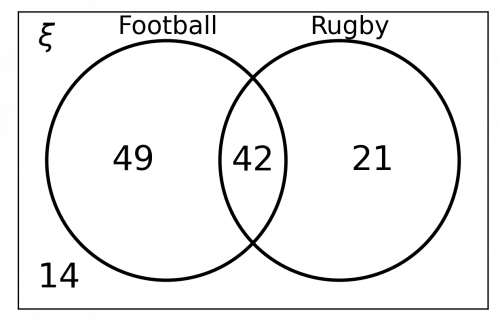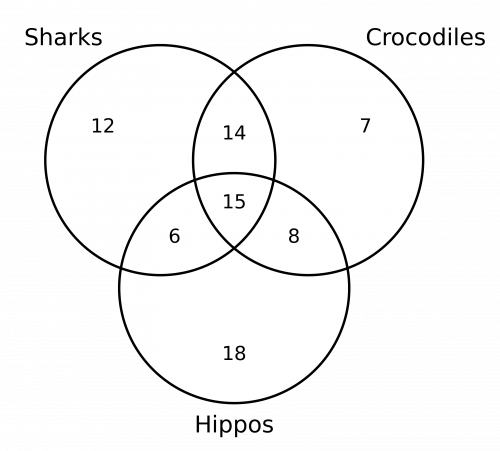# Venn Diagrams

GCSEKS3Level 4-5Level 6-7AQACambridge iGCSEEdexcelEdexcel iGCSEOCRWJEC

## Venn Diagrams

In this topic you will learn how to construct a Venn diagram and then how to use a Venn diagram to determine probabilities. Make sure you are happy with the following topics before continuing.

Level 4-5GCSEKS3AQAEdexcelOCRWJECCambridge iGCSEEdexcel iGCSE

## Drawing Venn Diagrams

A typical Venn diagram looks like the picture below. A Venn diagram is a way of grouping different parts of data known as sets. Drawing Venn diagrams is relatively simple as shown below.

Example: We have the numbers $\{1, 2, 3, 4, 5, 6, 7, 8, 9, 10, 11\}$.

Put the odd numbers in $A$ and prime numbers in $B$. Draw a Venn diagram for this information.

Step 1: Identify numbers that are odd: $\{1, \, 3, \, 5, \, 7, \, 9, \, 11 \}$ and numbers that are prime: $\{2, \, 3, \, 5, \, 7, \, 11 \}$. There’s some crossover here: $\{3, \, 5, \, 7, \, 11\}$ are contained in both, which means they go in the intersection.Step 2: Numbers that are odd but aren’t prime: $1$ and $9$. These will go in the part of section $A$ that doesn’t intersect with $B$.

Numbers that are prime but aren’t odd: $2$. These will go in the part of circle $B$ that doesn’t intersect with $A$.

Step 3: All the numbers that are neither odd nor prime: $4$, $6$, $8$, and $10$, will go inside the rectangle but outside either circle.

The result is the Venn diagram shown.

Level 4-5GCSEKS3AQAEdexcelOCRWJECCambridge iGCSEEdexcel iGCSE

## Interpreting Venn Diagrams

We can use Venn diagrams to solve probability problems.

Example: In the Venn diagram below, $A$ contains odd numbers and $B$ contains prime numbers.

A number is selected at random. Use the Venn diagram to determine the following:a) The number selected is both odd and prime.

Looking at the Venn diagram, we see that $4$ of the $11$ numbers are in both $A$ and $B$, so the probability of a number being both odd and prime is, $\dfrac{4}{11}$.

b) The number selected is odd and isn’t a prime number.

We are looking for all numbers that are in set $A$ but not set $B$, $1$ and $9$, which gives a probability of, $\dfrac{2}{11}$

Level 4-5GCSEKS3AQAEdexcelOCRWJECCambridge iGCSEEdexcel iGCSE
Level 6-7GCSEAQAEdexcelOCRWJECCambridge iGCSEEdexcel iGCSE

## Venn Diagrams and Conditional Probability

Venn diagrams can also be used to solve conditional probability problems.

Example: In the Venn diagram below, $G$ represents students selecting Geography and $H$ represents students selecting History. Use the Venn diagram to determine $\text{P}(G \text{ given } H)$ (Also written $\text{P}(G|H)$).We want to find the probability that someone takes Geography given that we already know they take History. If we know someone takes History, then they must be one of the $2+12=14$ people. Then, there are $12$ out of those $14$ people who take Geography, or in other words

$\text{P}(G|H)=\dfrac{12}{14}$

Level 6-7GCSEAQAEdexcelOCRWJECCambridge iGCSEEdexcel iGCSE

## Venn Diagrams Example Questions

a)  The first thing we need to do is draw a rectangle labelled $\xi$ to represent all the year $9$ students.  Then we need to draw two circles which overlap, one labelled ‘football’ and the other labelled ‘rugby’.

There is some simple information which we can input immediately.  We are told that $14$ students do not play rugby or football, so we can write ‘$14$’ outside the circles, but still inside the rectangle.  We are also told that $42$ students play both sports, so we can write ‘$42$’ in the intersection of the two circles.

The only information which we need to complete is the number of students who play football only and the number of students who play rugby only.   Since there are $126$ students in total, of which $14$ play neither sport and of which $42$ play both sports, we can work out how many students play football or rugby only:

$126-42-14 = 70\text{ students}$

Now that we know that a total of $70$ students play only football or only rugby, we are now in a better position to work out exactly how many students fall into each category.  We are told that the ratio of the number of students who play football only to the number of students who play rugby only is $7 : 3$.  This means that $\frac{7}{10}$ of the $70$ students play football only and $\frac{3}{10}$ of the $70$ students play rugby only.  (We are dealing in tenths here because the sum of the ratio is $10$.).

The number of students who play football only can be calculated as follows:

$\dfrac{7}{10}\times70 = 49\text{ students}$

The number of students who play rugby only can be calculated as follows:

$\dfrac{3}{10}\times70 = 21\text{ students}$

Therefore we need to insert ‘$49$’ inside the football circle (but not in the intersection) and ‘$21$’ inside the rugby circle (but not in the intersection).

Our completed Venn diagram should be similar to the below:b)  We know that $21$ students play rugby only out of the total $126$ students.  We can write this as the following fraction:

$\dfrac{21}{126}$

This fraction can be simplified to:

$\dfrac{1}{6}$Gold Standard Education

a)  The number of students in year $10$ is the total of:

• All the students that study geography only ($40$)
• All the students that study history only ($52$)
• All the students that study both geography and history ($14$)
• All the students that study neither history nor geography ($36$)

$40+52+14+36=142\text{ students}$

b)  We know that there are $142$ students in total and, of these, $14$ study both history and geography.  We can express this as the following fraction:

$\dfrac{14}{142}$

This fraction can be simplified to:

$\dfrac{7}{71}$

(You could give the probability as a decimal or as a percentage (the question doesn’t state how the answer should be given), but since both the decimal ($0.0985….$) and the percentage ($9.85…\%$) have several decimal places, leaving the probability as a fraction would probably be the most sensible option here, but the decimal and percentage answers are not incorrect.)

c)  The phrase ‘given that the student selected does not study geography’ tells us that we need to work out how many students do not study geography.  The students that don’t study geography are those who study history only ($52$ students) or those who study neither subject ($36$ students).  The total that do not study geography is therefore $52+36 = 88$ students.

Of these $88$ students, we need to find how many do not study history.  The $36$ that study neither subject are the ones that do not study history, so $36$ out of the $88$ who do not study geography do not study history.  We can write this as the following fraction:

$\dfrac{36}{88}$

This fraction can be simplified to:

$\dfrac{18}{44}$

This fraction can be simplified again to:

$\dfrac{9}{22}$

(The decimal answer of $0.409…..$ or the percentage answer of $40.909….\%$ are both acceptable ways to express the probability also.)Gold Standard Education

a)  What need to input the data.  Some of the data we are going to input will be easy, other parts may be more confusing.  Let’s start with one fact at a time:

• All $\bf{80}$ students like at least $\bf{1}$ of the animals.  There is not much we can do with this information.  However, this is a key piece of information since it tells us that once we have filled in the Venn diagram, the numbers should add up to $80$.

• $\bf{15}$ students like all $\bf{3}$ animals.  If $15$ students like all $3$ animals, then we can insert ‘$15$‘ in the intersection of all $3$ circles.

• $\bf{14}$ students like sharks and crocodiles but do not like hippos.  With this information we can insert ‘$14$’ in the section where sharks and crocodiles intersect (which should be outside the hippos circle).

• $\bf{23}$ students like crocodiles and hippos.  This is the piece of information which can cause confusion.  Although that statement says that $23$ students like crocodiles and hippos, this doesn’t mean that $23$ students like crocodiles and hippos only.  This figure of $23$ also includes students who like sharks as well as crocodiles and hippos.  This is the slightly confusing part of the question!  $15$ students like sharks, crocodiles and hippos, so that means that these $15$ students definitely like crocodiles and hippos.  So, if there are $23$ students in total who like crocodiles and hippos, that means that there are $8$ students who like crocodiles and hippos only.  Therefore we can now insert ‘$8$’ in the intersection of crocodiles and hippos (but not in the intersection of sharks, crocodiles and hippos).  You may need to read this paragraph a few times: it will sink in and make sense eventually!

• $\bf{21}$ students like sharks and hippos.  This is a very similar piece of information to the above piece of information about crocodiles and hippos.  $15$ students like sharks, crocodiles and hippos, therefore that means that there are at least $15$ students who like sharks and hippos.  Since we have been told that the total number of students who like sharks and hippos is $21$, then this means that $6$ students like sharks and hippos, but not crocodiles.  Therefore we can insert ‘$6$’ in the intersection of sharks and hippos.

• $\bf{44}$ students like crocodiles.  We know that $14$ students like crocodiles and sharks, that $15$ students like sharks, crocodiles and hippos, and that a further $8$ that like crocodiles and hippos, so if we add these numbers up we know that $37$ students like crocodiles.  Since we are told that $44$ students like crocodiles in total, this means that there are $7$ students who like crocodiles only.  Therefore we can insert ‘$7$’ in the part of the crocodile circle that does not overlap with any other section.

• $\bf{12}$ students only like sharks.  All we need to do is insert ‘$12$’ in the sharks only section.

All we need to do is work out how many students only like hippos.  We know that there are $80$ students in total, and we have filled in every section of the Venn diagram except this final hippos only section.  If we subtract all of the numbers from our total of $80$, we will be able to work out how many students only like hippos:

$80-12-14-7-6-15-8=18$

Therefore our completed Venn diagram will look similar to the below:b)  There are $80$ students in total, of which $18$ only like hippos.  This means that $18$ out of the $80$ only like hippos which can be written as the following fraction:

$\dfrac{18}{80}$

This fraction can be simplified to:

$\dfrac{9}{40}$

So, the probability of a person being selected at random and only liking hippos is $\frac{9}{40}$ (or $9\div40=0.225$ if you prefer to give your answer as a decimal.  The answer of $22.5\%$ is also acceptable.)

c)  There are $44$ students in total that like crocodiles.  There are $14$ students that like crocodiles and sharks and $8$ students that like crocodiles and hippos, so there are $22$ students that like crocodiles and one other animal.

This means that $22$ out of the $80$ only like crocodiles and one other type of animal, which can be written as the following fraction:

$\dfrac{22}{80}$

This fraction can be simplified to:

$\dfrac{11}{40}$

So, the probability of a person being selected at random and only liking hippos is $\frac{11}{40}$ or $11\div40=0.275$ if you prefer to give your answer as a decimal.  The answer of $27.5\%$ is also acceptable.Gold Standard Education

## Venn Diagrams Worksheet and Example Questions

### (NEW) Venn Diagrams Exam Style Questions - MME

Level 4-5Level 6-7GCSENewOfficial MME

## Venn Diagrams Drill Questions

### Venn Diagrams 2Gold Standard Education

Level 1-3GCSEKS3

Level 1-3GCSEKS3